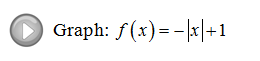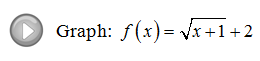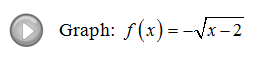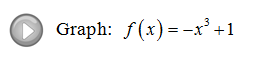## Pages

### Graphing Functions using Transformations

One way to graph functions is to simply plot points.  In this section, we cover a method used to quickly sketch graphs related to some basic functions. Here we focus on rigid transformations, that is, transformations that do not change the shape of the graph.

Vertical Translations: [ Interactive Graph ]
If k is any positive real number then,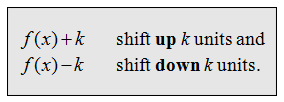The graph of the basic function f(x) = sqrt(x) follows:Using this basic graph and the vertical translations described above we can sketch f(x) = sqrt(x) + 2 by shifting all of the points up 2 units. Similarly, graph g(x) = sqrt(x 3 by shifting all points down 3 units.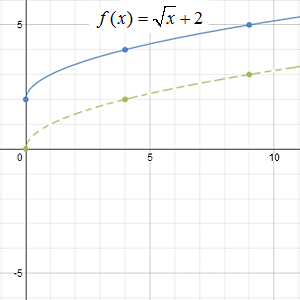Horizontal Translations:  [ Interactive Graph ]
If h is any positive real number then,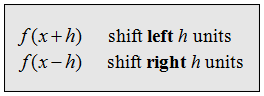Using the graph of f(x) = sqrt(x) and the horizontal translations described above we can sketch f(x) = sqrt(x + 4) by shifting all of the points left 4 units. Similarly, graph g(x) = sqrt( 3) by shifting all points right 3 units.Reflections: [ Interactive Graph ]
Given any function f(x),Using the graph of f(x) = sqrt(x) sketch the graph of f(x) = −sqrt(x)  by reflecting all of the points about the x-axis. Similarly, graph f(x) = sqrt(−x) by reflecting all of the points about the y-axis.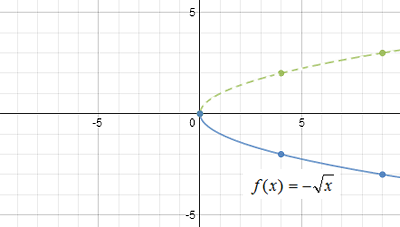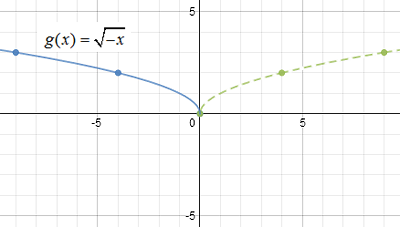For the first function f(x) = −sqrt(x) all of the y-values are negative which results in a reflection about the x-axis. For the second function f(x) = sqrt(−x) all of the x-values must be negative thus resulting in a reflection about the y-axis.
Sketch the graph.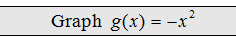The -1 indicates a reflection of the graph of the squaring function  f(x) = x^2 about the x-axis. Be sure to graph the squaring function using a dashed curve because it will be used as a guide and is not the answer. Next, reflect all points about the x-axis and draw in the final graph with a solid curve.General Steps for Graphing Functions using Transformations:
1. Identify and graph the basic function using a dashed curve.
2. Identify any reflections first and sketch them using the basic function as a guide.
3. Identify any translations.
4. Use this information to sketch the final graph using a solid curve.

Graph the function and determine the domain and range.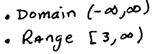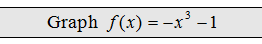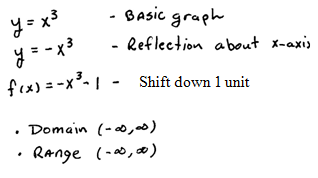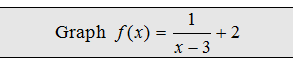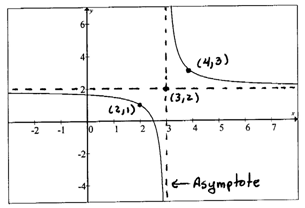Use the transformations to determine the equation that represents the given function.

Example: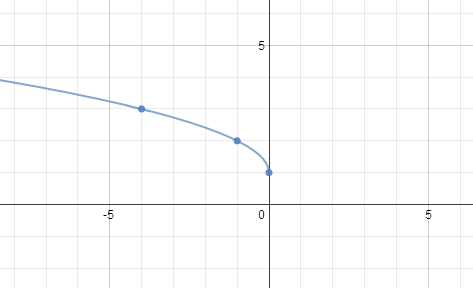From the general shape of the graph we can determine the basic function and transformations. The graph has the shape of the square root function,
y = sqrt(x)
Next, notice the reflection about the y-axis,
y = sqrt(x)
And finally, we see a shift up 1 unit.
y = sqrt(x) + 1Example:The given function has the general shape of the squaring function (parabola),
y = x^2
Next, notice the shift right 3 units,
y = ( 3)^
And finally, we see a shift down 2 units.
y = ( 3)^ 2Example:The given function has the general shape of the absolute value function,
y = abs(x)
Next, notice the reflection about the x-axis,
y = abs(x
Finally we see a shift left 1 unit and down 2 units
y = abs(x+1) − 2You Tube Videos: